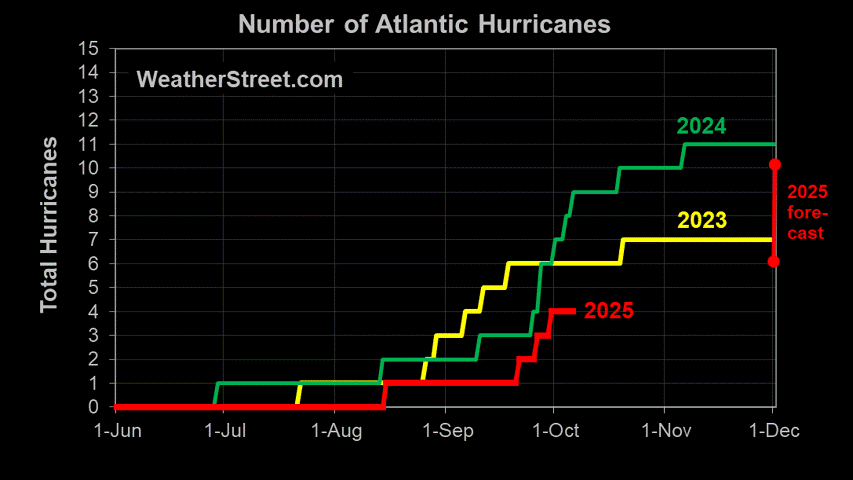# Gulf Coast Hurricane Tracker

A single source reference on tropical weather predictions. With a traditional focus on the upper Texas and Louisiana Gulf Coast we've maintained links to track all Atlantic Basin, Caribbean and eastern Pacific storm systems. We are now expanding our view to tropical storms throughout the world intending to be a comprehensive global storm tracking resource.## Sunday, August 23, 2009

### TSR Storm Alert - Hurricane BILL

Storm Alert issued at 23 Aug, 2009 21:00 GMT

Hurricane BILL is forecast to strike land to the following likelihood(s) at the given lead time(s):

probability for CAT 1 or above is 100% currently
probability for TS is 100% currently
St. Pierre and Miquelon
probability for CAT 1 or above is 55% within 9 hours
probability for TS is 100% within 9 hours
Sydney (46.1 N, 60.1 W)
probability for CAT 1 or above is 90% within 9 hours
probability for TS is 100% currently

Scotland
probability for TS is 55% in about 69 hours
Ireland
probability for TS is 55% in about 69 hours
Northern Ireland
probability for TS is 55% in about 69 hours
Grand Falls (48.6 N, 55.4 W)
probability for CAT 1 or above is 10% within 9 hours
probability for TS is 100% within 9 hours
St John's (47.6 N, 52.7 W)
probability for TS is 100% within 9 hours
Halifax (44.6 N, 63.6 W)
probability for TS is 75% currently
Charlottetown (46.2 N, 63.1 W)
probability for TS is 70% currently
Ullapool (58.0 N, 5.2 W)
probability for TS is 55% in about 69 hours
Portree (57.5 N, 6.2 W)
probability for TS is 55% in about 69 hours
Inverness (57.3 N, 4.3 W)
probability for TS is 55% in about 69 hours
Oban (56.3 N, 5.5 W)
probability for TS is 55% in about 69 hours
Glasgow (55.9 N, 4.3 W)
probability for TS is 55% in about 69 hours
Stranraer (55.0 N, 5.0 W)
probability for TS is 55% in about 69 hours
Ardara (54.8 N, 8.4 W)
probability for TS is 55% in about 69 hours
Belfast (54.6 N, 5.9 W)
probability for TS is 55% in about 69 hours
Sligo (54.3 N, 8.4 W)
probability for TS is 55% in about 69 hours
Belmullet (54.2 N, 10.0 W)
probability for TS is 55% in about 69 hours
Galway (53.3 N, 9.1 W)
probability for TS is 55% in about 69 hours
Dingle (52.2 N, 10.2 W)
probability for TS is 55% in about 69 hours

England
probability for TS is 50% in about 69 hours
the Isle of Man
probability for TS is 50% in about 69 hours
Wales
probability for TS is 45% in about 69 hours
Stornoway (58.3 N, 6.4 W)
probability for TS is 50% in about 69 hours
Dundee (56.5 N, 3.0 W)
probability for TS is 50% in about 69 hours
Edinburgh (55.8 N, 3.1 W)
probability for TS is 50% in about 69 hours
Workington (54.6 N, 3.4 W)
probability for TS is 50% in about 69 hours
Dublin (53.3 N, 6.3 W)
probability for TS is 50% in about 69 hours
Bantry (51.7 N, 9.4 W)
probability for TS is 50% in about 69 hours
Wick (58.5 N, 3.1 W)
probability for TS is 45% in about 69 hours
Aberdeen (57.2 N, 2.1 W)
probability for TS is 45% in about 69 hours
probability for TS is 45% in about 69 hours
Cork (51.9 N, 8.5 W)
probability for TS is 45% in about 69 hours
Kirkwall (59.0 N, 3.0 W)
probability for TS is 40% in about 69 hours
Newcastle (55.0 N, 1.6 W)
probability for TS is 40% in about 69 hours
Wexford (52.3 N, 6.5 W)
probability for TS is 40% in about 69 hours
York (54.2 N, 1.5 W)
probability for TS is 35% in about 69 hours
Manchester (53.5 N, 2.3 W)
probability for TS is 35% in about 69 hours

Note that
Red Alert (Severe) is CAT 1 or above to between 31% and 100% probability.
Yellow Alert (Elevated) is CAT 1 or above to between 10% and 30% probability, or TS to above 50% probability.
Green Alert (Low) is TS to between 31% and 50% probability.
CAT 1 means Hurricane strength winds of at least 74 mph, 119 km/h or 64 knots 1-min sustained.
TS means Tropical Storm strength winds of at least 39 mph, 63 km/h or 34 knots 1-min sustained.

For graphical forecast information and further details please visit http://www.tropicalstormrisk.com/

This alert is provided by Tropical Storm Risk (TSR) which is sponsored by UCL, Aon Benfield, Royal & SunAlliance, Crawford & Company and Aon Benfield UCL Hazard Research Centre. TSR acknowledges the support of the UK Met Office.

2010 Atlantic Hurricanes (courtesy of Weatherstreet.com)NOAA Gulf of Mexico Radar (courtesy of Weatherstreet.com)NOAA West Atlantic & Caribbean Radar (courtesy of Weatherstreet.com)NOAA East Atlantic Radar (courtesy of Weatherstreet.com)